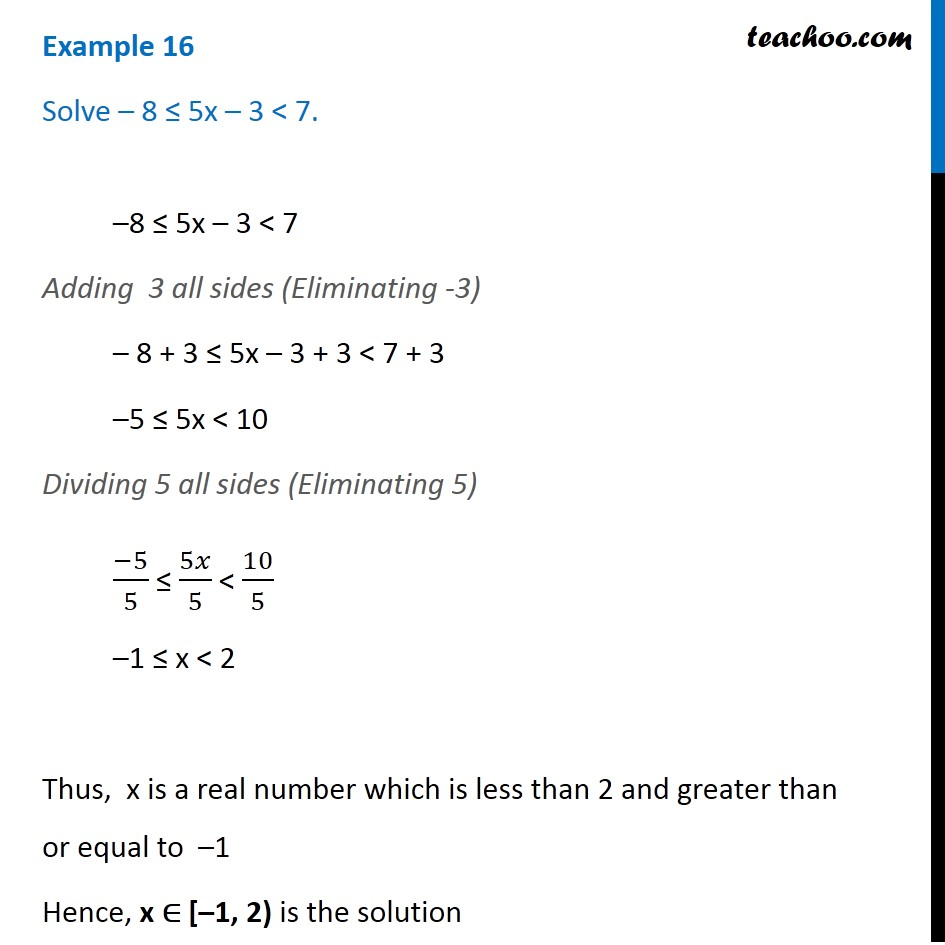Subscribe to our Youtube Channel - https://you.tube/teachoo

1. Chapter 6 Class 11 Linear Inequalities
2. Concept wise
3. Solving inequality (both sides)

Transcript

Example 16 Solve – 8 ≤ 5x – 3 < 7. –8 ≤ 5x – 3 < 7 Adding 3 all sides (Eliminating -3) – 8 + 3 ≤ 5x – 3 + 3 < 7 + 3 –5 ≤ 5x < 10 Dividing 5 all sides (Eliminating 5) (−5)/5 ≤ 5𝑥/5 < 10/5 –1 ≤ x < 2 Thus, x is a real number which is less than 2 and greater than or equal to –1 Hence, x ∈ [–1, 2) is the solution

Solving inequality (both sides)